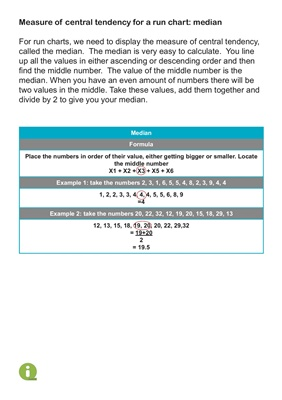Measure of central tendency for a run chart: median

For run charts, we need to display the measure of central tendency,

called the median. The median is very easy to calculate. You line

up all the values in either ascending or descending order and then

find the middle number. The value of the middle number is the

median. When you have an even amount of numbers there will be

two values in the middle. Take these values, add them together and

divide by 2 to give you your median.

Median

Formula

Place the numbers in order of their value, either getting bigger or smaller. Locate

the middle number

X1 + X2 + X3 + X5 + X6

Example 1: take the numbers 2, 3, 1, 6, 5, 5, 4, 8, 2, 3, 9, 4, 4

1, 2, 2, 3, 3, 4, 4, 4, 5, 5, 6, 8, 9

=4

Example 2: take the numbers 20, 22, 32, 12, 19, 20, 15, 18, 29, 13

12, 13, 15, 18, 19, 20, 20, 22, 29,32

= 19+20

2

= 19.5